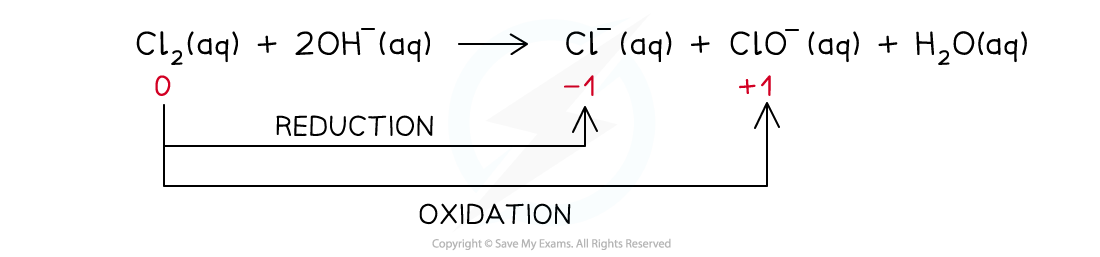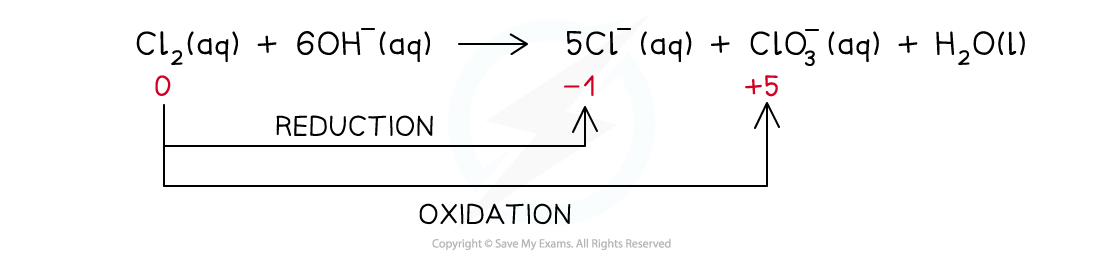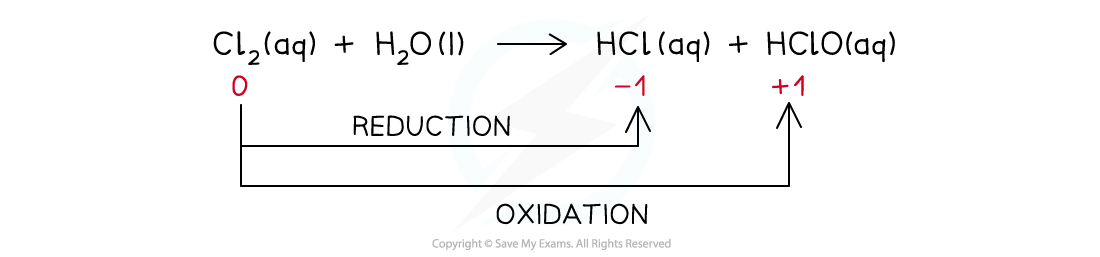# Edexcel A Level Chemistry:复习笔记2.3.3 Halogen Redox Reactions

### Halogen Redox Reactions

#### Reactions with Group 1 & 2 metals

• The halogens react with some metals to form ionic compounds which are metal halide salts
• In all reactions where halogens are reacting with metals, the metals are being oxidised
• Reaction of sodium and chlorine
• 2Na (s) + Cl2 (g) → 2NaCl (s)
• Na is being oxidised, the oxidation number is changing from 0 to +1

• Calcium is a group 2 metal:
• Ca (s) + Br2 (l) → CaBr2 (s)
• Ca is being oxidised, the oxidation number is changing from 0 to +2

• Therefore the halogens are acting as oxidising agents

#### Reactions with Iron(II)

• Chlorine and bromine can oxidise iron(II) to iron(III)

Cl2 (g) + 2Fe2+ (aq) → 2Cl- (aq) + 2Fe3+ (aq)

Br2 (g) + 2Fe2+ (aq) → 2Br- (aq) + 2Fe3+ (aq)

• However, iodine is not a strong enough reducing argent to oxidise iron(II) to iron(III)
• Iodine is actually oxidised from iodide ions to iodine by iron(III)

2I- (aq) + 2Fe3+ (aq) → I2 (aq) + 2Fe2+ (aq)

#### Disproportionation reaction

• A disproportionation reaction is a reaction in which the same species is both oxidised and reduced
• The reaction of chlorine with dilute alkali is an example of a disproportionation reaction
• In these reactions, the chlorine gets oxidised and reduced at the same time
• Different reactions take place at different temperatures of the dilute alkali

#### Chlorine in cold alkali (15 oC)

• The reaction that takes place is:• The ionic equation is:• The ionic equation shows that the chlorine gets both oxidised and reduced
• Chlorine gets oxidised as there is an increase in ox. no. from 0 to +1 in ClO-(aq)
• The half-equation for the oxidation reaction is:• Chlorine gets reduced as there is a decrease in ox. no. from 0 to -1 in Cl-(aq)
• The half-equation for the reduction reaction is:#### Chlorine in hot alkali (70 oC)

• The reaction that takes place is:• The ionic equation is:• The ionic equation shows that the chlorine gets both oxidised and reduced
• Chlorine gets oxidised as there is an increase in ox. no. from 0 to +5 in ClO3-(aq)
• The half-equation for the oxidation reaction is:• Chlorine gets reduced as there is a decrease in ox. no. from 0 to -1 in Cl-(aq)
• The half-equation for the reduction reaction is:#### Drinking water

• Chlorine can be used to clean water and make it drinkable
• The reaction of chlorine in water is a disproportionation reaction in which the chlorine gets both oxidised and reducedThe disproportionation reaction of chlorine with water in which chlorine gets reduced to HCl and oxidised to HClO

• Chloric(I) acid (HClO) sterilises water by killing bacteria
• Chloric acid can further dissociate in water to form ClO-(aq):

HClO (aq) → H+ (aq) + ClO- (aq)

• ClO-(aq) also acts as a sterilising agent cleaning the water